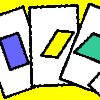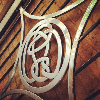Filter by: Content type:
Age range:
Challenge level:

### There are 23 results### Triangle Transformation

##### Age 7 to 14Challenge Level

Start with a triangle. Can you cut it up to make a rectangle?### Dividing the Field

##### Age 14 to 16Challenge Level

A farmer has a field which is the shape of a trapezium as illustrated below. To increase his profits he wishes to grow two different crops. To do this he would like to divide the field into two. . . .##### Age 11 to 14Challenge Level

We started drawing some quadrilaterals - can you complete them?### Trapezium Four

##### Age 14 to 16Challenge Level

The diagonals of a trapezium divide it into four parts. Can you create a trapezium where three of those parts are equal in area?##### Age 11 to 14Challenge Level

Four rods, two of length a and two of length b, are linked to form a kite. The linkage is moveable so that the angles change. What is the maximum area of the kite?##### Age 14 to 16Challenge Level##### Age 11 to 14Challenge Level

A game for 2 or more people, based on the traditional card game Rummy.### Poly Plug Rectangles

##### Age 5 to 14Challenge Level

The computer has made a rectangle and will tell you the number of spots it uses in total. Can you find out where the rectangle is?### Opposite Vertices

##### Age 11 to 14Challenge Level

Can you recreate squares and rhombuses if you are only given a side or a diagonal?### Shapely Pairs

##### Age 11 to 14Challenge Level

A game in which players take it in turns to turn up two cards. If they can draw a triangle which satisfies both properties they win the pair of cards. And a few challenging questions to follow...### Property Chart

##### Age 11 to 14Challenge Level

A game in which players take it in turns to try to draw quadrilaterals (or triangles) with particular properties. Is it possible to fill the game grid?### Lawnmower

##### Age 14 to 16Challenge Level

A kite shaped lawn consists of an equilateral triangle ABC of side 130 feet and an isosceles triangle BCD in which BD and CD are of length 169 feet. A gardener has a motor mower which cuts strips of. . . .##### Age 14 to 16Challenge Level

Investigate the properties of quadrilaterals which can be drawn with a circle just touching each side and another circle just touching each vertex.### Areas of Parallelograms

##### Age 14 to 16Challenge Level

Can you find the area of a parallelogram defined by two vectors?##### Age 11 to 16

This gives a short summary of the properties and theorems of cyclic quadrilaterals and links to some practical examples to be found elsewhere on the site.### Long Short

##### Age 14 to 16Challenge Level

What can you say about the lengths of the sides of a quadrilateral whose vertices are on a unit circle?##### Age 14 to 16Challenge Level

The points P, Q, R and S are the midpoints of the edges of a non-convex quadrilateral.What do you notice about the quadrilateral PQRS and its area?##### Age 14 to 16Challenge Level

A picture is made by joining five small quadrilaterals together to make a large quadrilateral. Is it possible to draw a similar picture if all the small quadrilaterals are cyclic?##### Age 14 to 16Challenge Level

Four rods are hinged at their ends to form a convex quadrilateral. Investigate the different shapes that the quadrilateral can take. Be patient this problem may be slow to load.##### Age 14 to 16Challenge Level

Explore when it is possible to construct a circle which just touches all four sides of a quadrilateral.##### Age 11 to 14Challenge Level

How many questions do you need to identify my quadrilateral?### An Equilateral Triangular Problem

##### Age 11 to 14Challenge Level

Take an equilateral triangle and cut it into smaller pieces. What can you do with them?### Making Rectangles, Making Squares

##### Age 11 to 14Challenge Level

How many differently shaped rectangles can you build using these equilateral and isosceles triangles? Can you make a square?